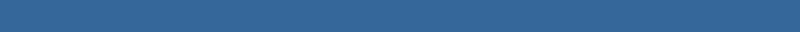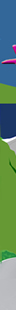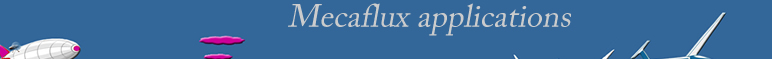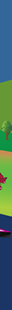Video tutorials and tutorials software MECAFLUX

Video tutorials and presentations of fluid mechanics software

The list of functions is detailed on page: functions software Mecaflux Standard.

• Presentation software mecaflux standard and its various tools fluid mechanics calculations

Calculations of losses and networks:

• You will learn in this tutorial how to choose a suitable diameter pipe at the desired rate by comparing losses linear loads of different diameters series of pipes commonly used.(fr)

• In this tutorial (below) you will learn very quickly to compute the pressure drop of a regular straight duct depending on the flow rate, the roughness and the fluid chosen (air, water, .... a database allows you to choose the temperature and the desired fluid)(fr)

• In this tutorial (below) you will learn very quickly to compute the singular pressure loss versus flow, and selected accessories (bends, cones, pipe, exits tanks ...)(fr)

• Tutorials 3 parts design pumping system based on a school ordeal ELECTROTECHNIQUE:

• The tutorial (below) teaches you how to add a new element in the singular pressure loss chart from a chart table of coefficients of singular pressure loss statement for example in Idel'Cik (memento head loss)(fr)

• The tutorial (below) will explain how to plot a graph of the evolution pressure drop of your system for a range of flow rates.(fr)

• The tutorial (below) shows how to use the pressure losses that you calculated to find the operating flow rate of the pump that you connect to your network.(fr)

• The tutorial (below) shows how to use the pressure losses that you calculated to predict the production of your turbine installation.(fr)

• The tutorial (below) shows how to use the editor to choose your fluid and its parameters according to its temperature ...(fr)

Calculations Lift and Drag profiles:

• The tutorial (below) shows how to use the tab for calculating drag and lift to quantify the effects of fluid flow on an object of various forms(fr)

• The tutorial (below) shows how to use the tab for calculating drag and lift to quantify the effects of fluid flow on an object of various forms......(fr)

• The tutorial (below) shows how to use the profile editor to calculate the polar Profile. (fr)